# What is interesting about the normal distribution

## Normal distribution / Gaussian bell curve

The normal distribution is probably the most frequently used distribution in statistics. On the one hand, this is due to the fact that you can describe the realizations of many scientific, technical and economic variables quite well using the normal distribution; on the other hand, the Central Limit Theorem states that the mean value of n independent, identically distributed random variables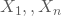for large n converge to the normal distribution, regardless of the distribution of the.

### Properties of normal distribution

The normal distribution is often also called the Gaussian distribution or Gaussian bell curve, as it was largely analyzed by the mathematician Carl-Friedrich Gauß and its density function has a bell shape. The density function is symmetrical and also has the two parameters mean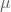and variance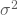.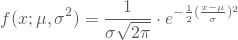If you integrate the density function, you get the associated distribution function: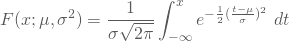### Visualization of the normal distribution

Graphically, the density function of the normal distribution results in a bell like the one on the left: This shape shows that deviations from the mean value upwards or downwards are the less likely the further they are from the mean value; the graphic on the right shows the associated distribution function: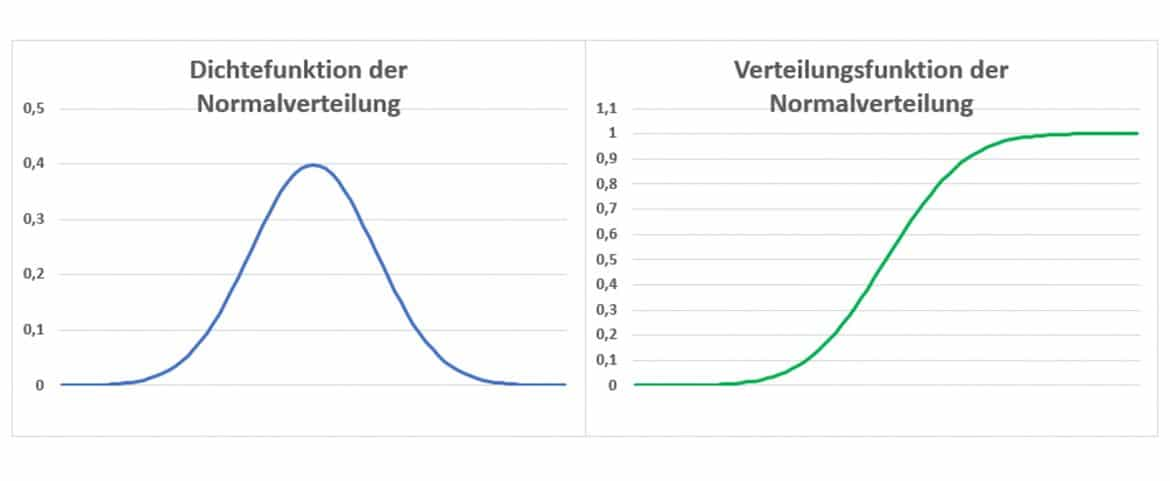The larger the mean value of the respective distribution, the further the density and distribution functions move to the right; the greater the variance, the wider both are.

### Special case: standard normal distribution

The standard normal distribution with mean is a special case that is frequently used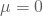and variance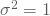into which you can convert any normal distribution by standardizing x into z. To do this, you subtract the meanof your x and divide the difference by the standard deviation, the root of the variance.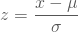Incidentally, the standard normal distribution is tabulated and many parametric estimation and test procedures use it.

### Application of the normal distribution

For example, a machine is supposed to pack sugar in packages of 1kg. The filling weight is normally distributed with the mean valueand also has a variance of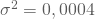. You would then like to know the probability with which a maximum weight of 1.010 kg will be realized. To do this, you standardize your x-value in z and determine the value of the distribution function of the standard normal distribution at this point: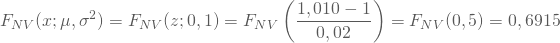You therefore get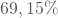Your sugar packets with a maximum weight of 1.01 kg.

In contrast, you can determine which weight has a probability of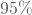is not exceeded. To do this, look in the table to see which z-value for the distribution function at that point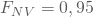heard and found the value z = 1.645. After changing the above standardization formula, you will get: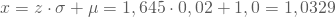With a probability ofthe filling weight of a package does not exceed 1.0329 kg.

Because of the symmetry of the normal distribution, the following applies: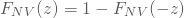or.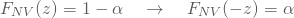With this you can easily determine the value from your above result, which only has a probability ofis fallen below. You get -z = -1.645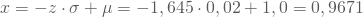With a probability ofthe filling weight is below 0.9671 kg.

It is also interesting what percentage of the realizations of the random variables are at most a distance equal to the multiple of the standard deviation from the mean: The following applies here for any normal distribution: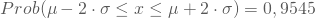and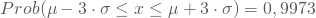This allows you to make initial estimates of your distribution very easily.

For your example and twice the standard deviation result as interval limits within which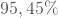the realizations are accordinglyWeigh with itthe parcels therefore at least 960 g and at most 1040 g. The manufacturer should, however, think about setting the machine again so that there is less fluctuation.

In the event that certain conditions exist, especially for large sample sizes n, you can approximate other distributions using the very easy-to-use normal distribution: